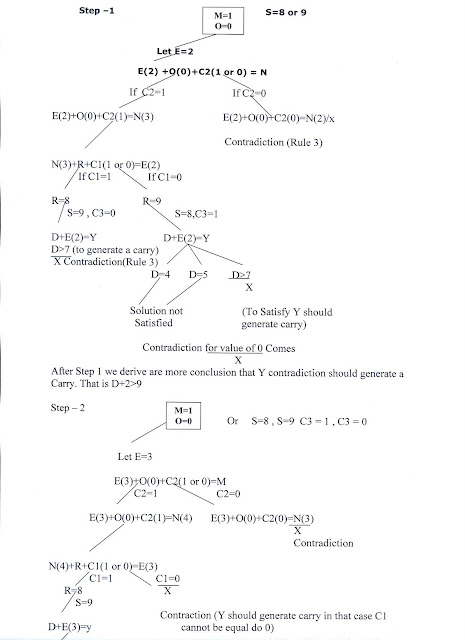Friday, July 23, 2010

Constraint Satisfaction Problem1.

CONSTRAINT SATISFACTION:-

Many problems in AI can be considered as problems of constraint satisfaction, in which the goal state satisfies a given set of constraint. constraint satisfaction problems can be solved by using any of the search strategies. The general form of the constraint satisfaction procedure is as follows:

Until a complete solution is found or until all paths have led to lead ends, do

1. select an unexpanded node of the search graph.

2. Apply the constraint inference rules to the selected node to generate all possible new constraints.

3. If the set of constraints contains a contradiction, then report that this path is a dead end.

4. If the set of constraints describes a complete solution then report success.

5. If neither a constraint nor a complete solution has been found then apply the rules to generate new partial solutions. Insert these partial solutions into the search graph.

Example: consider the crypt arithmetic problems.

SEND
+ MORE
----------
MONEY
----------

Assign decimal digit to each of the letters in such a way that the answer to the problem is correct to the same letter occurs more than once , it must be assign the same digit each time . no two different letters may be assigned the same digit. Consider the crypt arithmetic problem.

SEND
+ MORE
-----------
MONEY
-----------

CONSTRAINTS:-

1. no two digit can be assigned to same letter.

2. only single digit number can be assign to a letter.

1. no two letters can be assigned same digit.

2. Assumption can be made at various levels such that they do not contradict each other.

3. The problem can be decomposed into secured constraints. A constraint satisfaction approach may be used.

4. Any of search techniques may be used.

5. Backtracking may be performed as applicable us applied search techniques.

6. Rule of arithmetic may be followed.

Initial state of problem.
D=?
E=?
Y=?
N=?
R=?
O=?
S=?
M=?
C1=?
C2=?
C1 ,C 2, C3 stands for the carry variables respectively.

Goal State: the digits to the letters must be assigned in such a manner so that the sum is satisfied.

Solution Process:

We are following the depth-first method to solve the problem.

1. initial guess m=1 because the sum of two single digits can generate at most a carry '1'.

2. When n=1 o=0 or 1 because the largest single digit number added to m=1 can generate the sum of either 0 or 1 depend on the carry received from the carry sum. By this we conclude that o=0 because m is already 1 hence we cannot assign same digit another letter(rule no.)

3. We have m=1 and o=0 to get o=0 we have s=8 or 9, again depending on the carry received from the earlier sum.

The same process can be repeated further. The problem has to be composed into various constraints. And each constraints is to be satisfied by guessing the possible digits that the letters can be assumed that the initial guess has been already made . rest of the process is being shown in the form of a tree, using depth-first search for the clear understandability of the solution process.D>6(Controduction)

1.ye to bilkul samajh me nhi aaya

2.Thanks for sharing information about Artificial Intelligence.
Artificial Intelligence Solutions

3.Impressive!Thanks for the post
Artificial intelligence Solutions

4.thanks , i found it better here. https://www.youtube.com/watch?v=W-uWft0Q3-M&t=56s

5.Thank You For Sharing this information.
Artificial intelligence Solutions